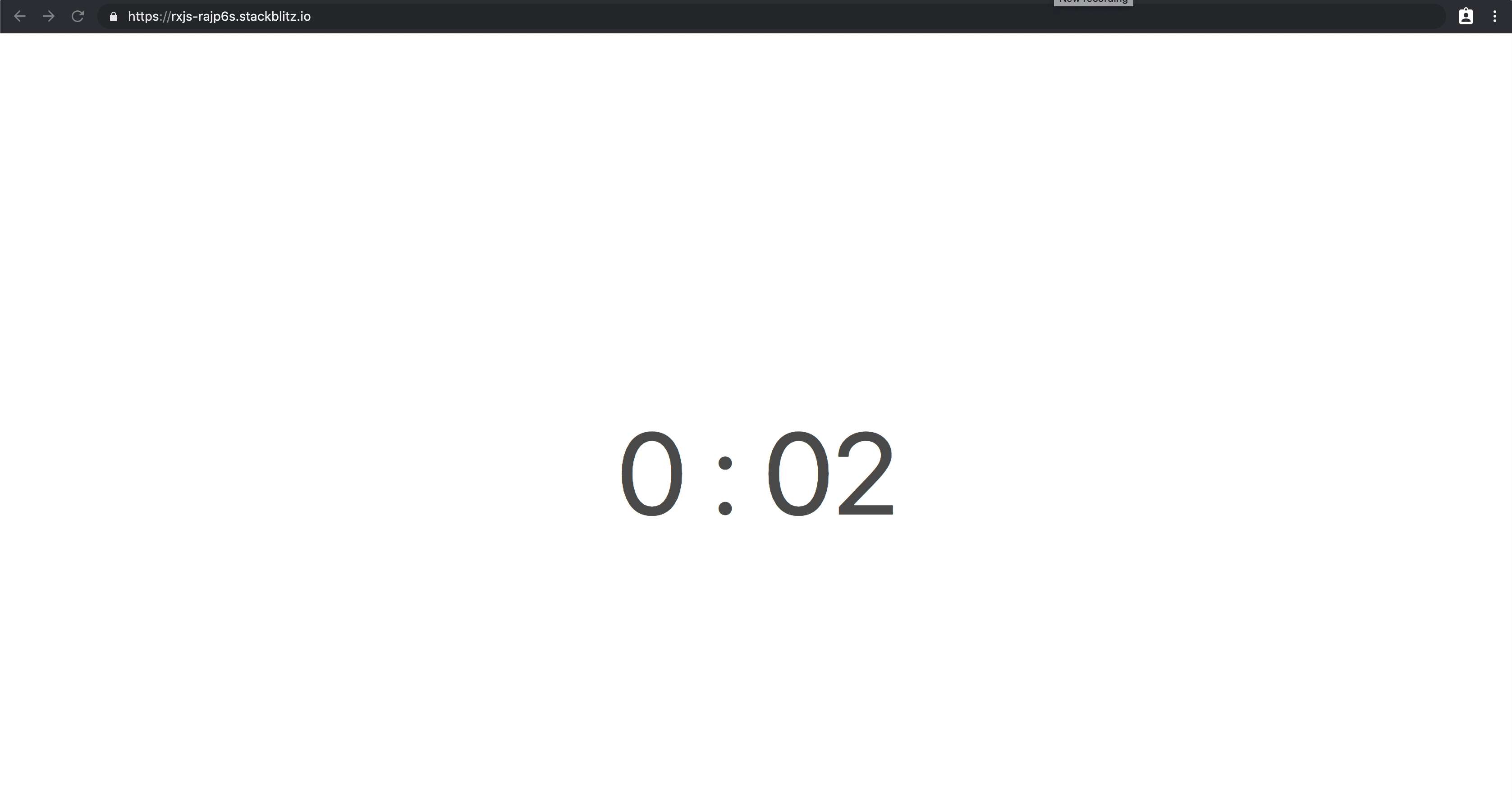# A simple Countdown with RxJS

### Preview

Before we get started, you can view the result using the awesome Stackblitz. You can see a preview of the final result at this link.

The timer starts automatically when you land on the page, you can click on the time to stop it, and click again to restart the timer.

When the time ends, the user will be prompted to take a break! It’s a very simple example, so the timer won’t restart.

### Constants

Let’s first define some of the constants we’re going to use:

• We define `K` as we’re going to use this a lot, as we will be dealing with milliseconds, so we assign `1000` as value
• The interval is the amount of time that needs to elapse in order to update the timer. If we set it to `5000`, the timer would be updated every 5 seconds
• We set the minutes we want our timer to be long and its time in milliseconds
``const K = 1000;const INTERVAL = K;const MINUTES = 25;const TIME = MINUTES * K * 60;``

### State variables

In order to keep the time’s state when pausing/resuming the timer, we define two variables:

``let current: number;let time = TIME;``
• `current` will be continually updated every second
• `time` will be updated when the timer stops

### Helper functions

We define some helper functions used by our streams. We want to:

• convert remaining time to milliseconds and seconds
• have functions to display remaining minutes and seconds
``const toMinutes = (ms: number) =>    Math.floor(ms / K / 60);const toSeconds = (ms: number) =>    Math.floor(ms / K) % 60;const toSecondsString = (ms: number) => {    const seconds = toSeconds(ms);    return seconds < 10 ? `0\${seconds}` : seconds.toString();}const toMs = (t: number) => t * INTERVAL;const currentInterval = () => time / INTERVAL;const toRemainingSeconds = (t: number) => currentInterval() - t;``

### Defining the Rx streams

First, we define the `timer\$` stream:

• we use the observable creator `timer`, that emits every `INTERVAL` times, which basically means it will emit every second

The stream will convert the milliseconds emitted from `timer` to the remaining seconds.

``const toggle\$ = new BehaviorSubject(true);const remainingSeconds\$ = toggle\$.pipe(    switchMap((running: boolean) => {        return running ? timer(0, INTERVAL) : NEVER;    }),    map(toRemainingSeconds),    takeWhile(t => t >= 0));``

Let’s explain detail what this does:

``````**toggle\$** -> true...false...true

-----

**switchMap** to:

**if toggle is true -> timer(0, INTERVAL = 1000)** -> 0...1000...2000
**if toggle is false ? ->** NEVER = do not continue

----

**map(toRemainingSeconds)** -> ms elapsed mapped to remaining seconds (ex. 1500)

----

**takeWhile(remainingSeconds)** -> complete once **remainingSeconds\$'s** value  is no more >= 0
``````

Let’s consider the operators used:

• the mapper `toSeconds` will convert the milliseconds returned by the observable to the number of seconds that are remaining
• by using the operator `takeWhile` we’re basically telling the `remainingSeconds\$` observable to keep going until the seconds remaining are greater or equal than 0
• After that, `remainingSeconds\$` will emit its completion callback that we can use to replace the timer with some other content

Before creating the relative minutes and seconds we will be displaying, we want to be able to stop and resume and timer.

If `toggle\$` is emitted with `true` as value, the timer keeps running, while if it gets emitted with `false` it will stop, as instead of mapping to `remainingSeconds\$` it will emit the observable `NEVER` .

### Pausing and resuming the timer

By using `fromEvent` , we can listen to click events and update the behavior subject by toggling its current value.

``const toggleElement = document.querySelector('.timer');fromEvent(toggleElement, ‘click’).subscribe(() => {    toggle\$.next(!toggle\$.value);});``

But `toggle\$` also does something else:

• every time the timer gets stopped, we want to update the time variable with the current time, so that the next time the timer restarts, it will restart from the current time.
``toggle\$.pipe(    filter((toggled: boolean) => !toggled)).subscribe(() => {    time = current;});``

Now, we can define the milliseconds observable we’re going to use to display minutes and seconds:

``const ms\$ = time\$.pipe(    map(toMs),    tap(t => current = t));``

Every time `ms\$` emits, we use the `tap` operator to update the stateful variable `current`.

Next, we’re going to define minutes and seconds by reusing the helper methods we defined earlier in the article.

``const minutes\$ = ms\$.pipe(    map(toMinutesDisplay),    map(s => s.toString()),    startWith(toMinutesDisplay(time).toString()));const seconds\$ = ms\$.pipe(    map(toSecondsDisplayString),    startWith(toSecondsDisplayString(time).toString()));``

And that’s it! Our streams are ready and can now update the DOM.

### Updating the DOM

We define a simple function called `updateDom` that takes an observable as the first argument and an HTML element as the second one. Every time the source emits, it will update the `innerHTML` of the node.

HTML:

``<div class="timer">    <span class="minutes"></span>    <span>:</span>    <span class="seconds"></span></div>``
``// DOM nodesconst minutesElement = document.querySelector('.minutes');const secondsElement = document.querySelector('.seconds');updateDom(minutes\$, minutesElement);updateDom(seconds\$, secondsElement);function updateDom(source\$: Observable<string>, element: Element) {    source\$.subscribe((value) => element.innerHTML = value);}``

Lastly, we want to display a message when the timer stops:

``timer\$.subscribe({    complete: () => updateDom(of('Take a break!'), toggleElement)});``You can find the complete code snippet on Stackblitz.

Hope you enjoyed the article and leave a message if you agree, disagree, or if you would do anything differently!